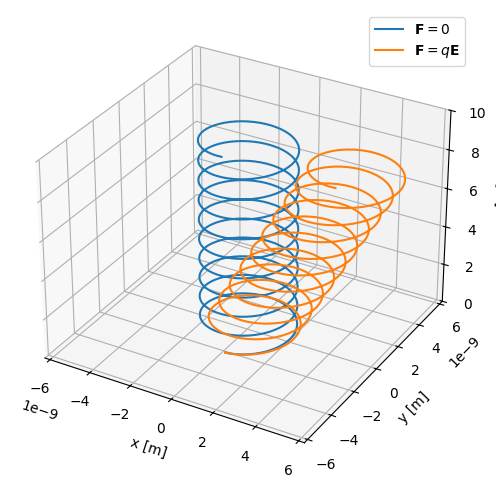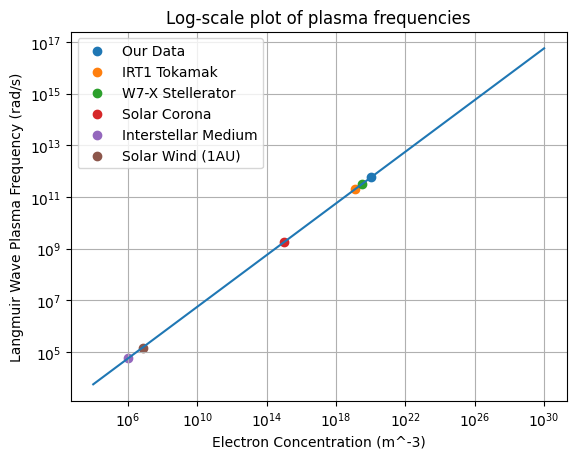# Length Plasma Parameters (plasmapy.formulary.lengths)

Functions to calculate fundamental plasma length parameters.

## Functions

 Debye_length(T_e, n_e) Calculate the characteristic decay length for electric fields, gyroradius(B, particle, *[, Vperp, T_i, T]) Return the particle gyroradius. inertial_length(n, particle) Calculate a charged particle's inertial length.

## Aliases

PlasmaPy provides short-named (alias) versions of the most common plasma functionality. These aliases are only given to functionality where there is a common lexicon in the community, for example has the alias . All aliases in PlasmaPy are denoted with a trailing underscore _.

 cwp_(n, particle) Alias to inertial_length. lambdaD_(T_e, n_e) Alias to Debye_length. rc_(B, particle, *[, Vperp, T_i, T]) Alias to gyroradius. rhoc_(B, particle, *[, Vperp, T_i, T]) Alias to gyroradius.

## Examples